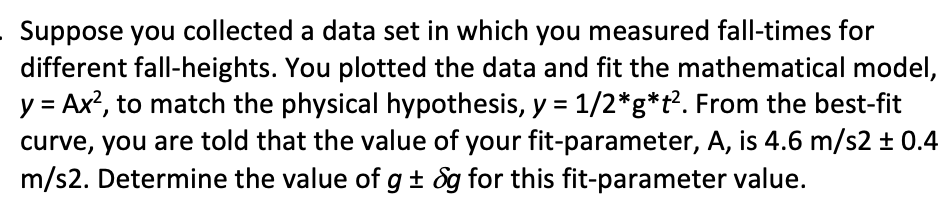# Suppose you collected a data set in which you measured fall-times fordifferent fall-heights. You plotted the data and fit the mathematical model,y Ax2, to match the physical hypothesis, y 1/2*g*t2. From the best-fitcurve, you are told that the value of your fit-parameter, A, is 4.6 m/s2 t 0.4m/s2. Determine the value of g+ &g for this fit-parameter value.

Question
135 views

Suppose you collected a data set in which you measured fall-times for different fall-heights. You plotted the data and fit the mathematical model, y = Ax2, to match the physical hypothesis, y = 1/2*g*t2. From the best-fit curve, you are told that the value of your fit-parameter, A, is 4.6 m/s2 ± 0.4 m/s2. Determine the value of g ± (delta)g for this fit-parameter value.help_outlineImage TranscriptioncloseSuppose you collected a data set in which you measured fall-times for different fall-heights. You plotted the data and fit the mathematical model, y Ax2, to match the physical hypothesis, y 1/2*g*t2. From the best-fit curve, you are told that the value of your fit-parameter, A, is 4.6 m/s2 t 0.4 m/s2. Determine the value of g+ &g for this fit-parameter value. fullscreen
check_circle

star
star
star
star
star
2 Ratings
Step 1

The relation between the fit parameter A from the given mathematical model to and the parameter g from the physical hypothesis from the problem above can be shown to be simply,

Step 2

From the calculated value of the fit parameter A , calculate g as,

Step 3

The uncertainty in g is c...

### Want to see the full answer?

See Solution

#### Want to see this answer and more?

Solutions are written by subject experts who are available 24/7. Questions are typically answered within 1 hour.*

See Solution
*Response times may vary by subject and question.
Tagged in

### Other